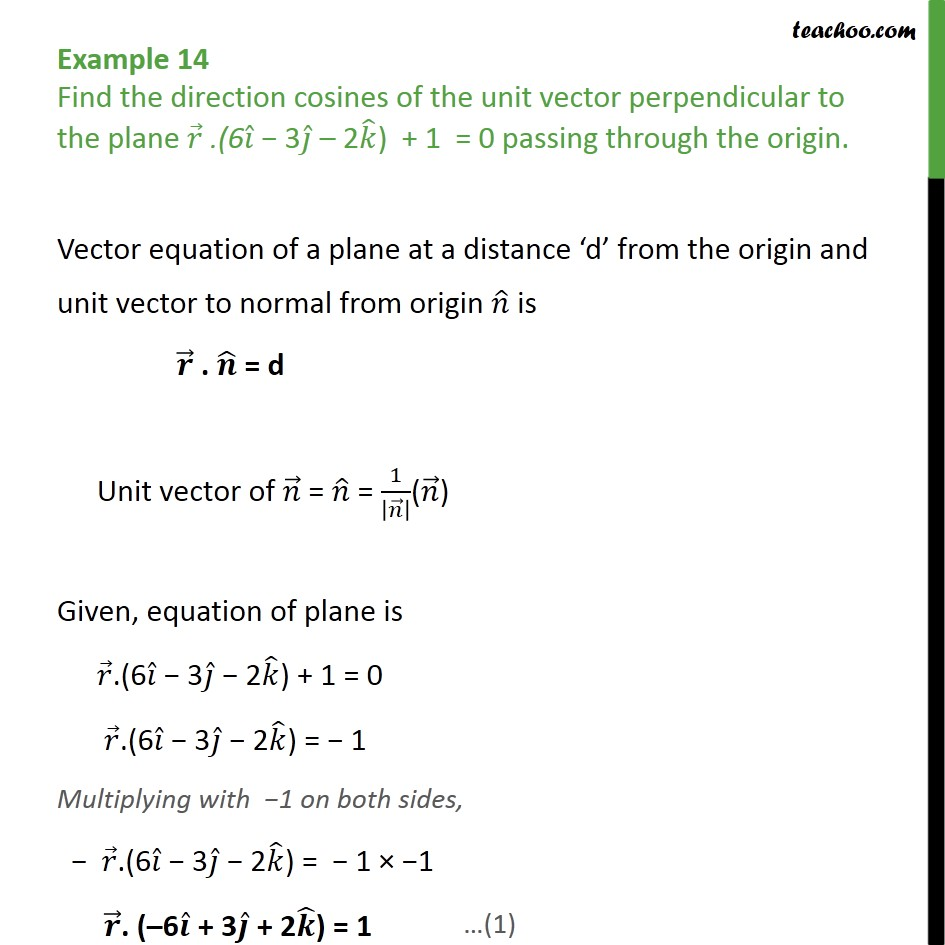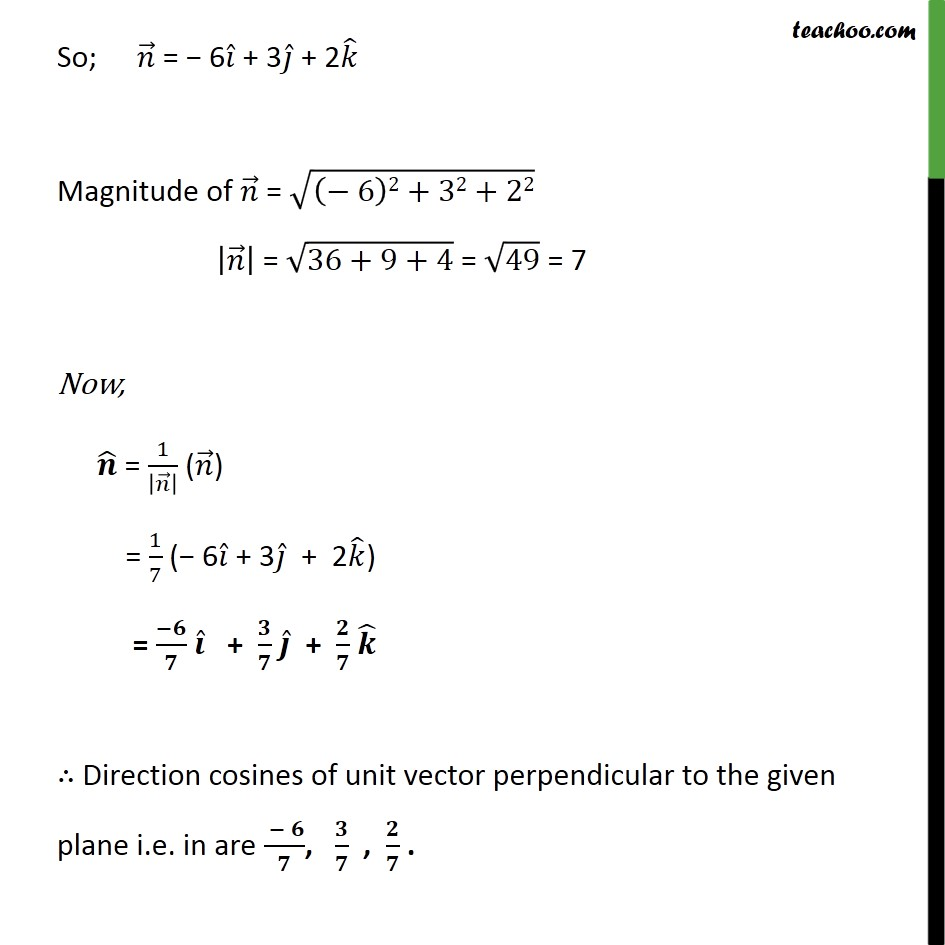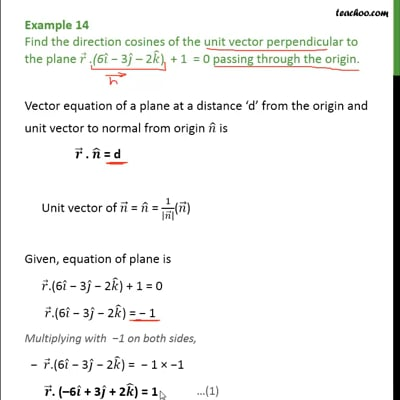Examples

Chapter 11 Class 12 Three Dimensional Geometry
Serial order wiseThis video is only available for Teachoo black users

Solve all your doubts with Teachoo Black (new monthly pack available now!)

### Transcript

Example 14 Find the direction cosines of the unit vector perpendicular to the plane .(6 3 2 ) + 1 = 0 passing through the origin. Vector equation of a plane at a distance d from the origin and unit vector to normal from origin is . = d Unit vector of = = 1 ( ) Given, equation of plane is .(6 3 2 ) + 1 = 0 .(6 3 2 ) = 1 Multiplying with 1 on both sides, .(6 3 2 ) = 1 1 . ( 6 + 3 + 2 ) = 1 So; = 6 + 3 + 2 Magnitude of = 6 2+32+22 = 36+9+4 = 49 = 7 Now, = 1 ( ) = 1 7 ( 6 + 3 + 2 ) = + + Direction cosines of unit vector perpendicular to the given plane i.e. in are , , .